• 前两篇博客介绍的是线性回归线性回归的一个问题是有可能出现欠拟合现象，解决欠拟合其中的一个方法是本文的多项式扩展，还有一个是后面的博客会介绍的局部加权线性回归（Locally Weighted Linear Regression，LWLR...


文章目录
概述代码分析总结

概述
前两篇博客介绍的是线性回归，线性回归的一个问题是有可能出现欠拟合现象，因为它求的是具有最小均方误差的无偏估计。显而易见，如果模型欠拟合将不能取得最好的预测效果。所以有些方法允许在估计中引入一些偏差，从而降低预测的均方误差。其中的一个方法是本文的多项式扩展，还有一个是后续的博客会介绍的局部加权线性回归（Locally Weighted Linear Regression，LWLR）。在正式介绍多项式扩展之前，先来看一张对比图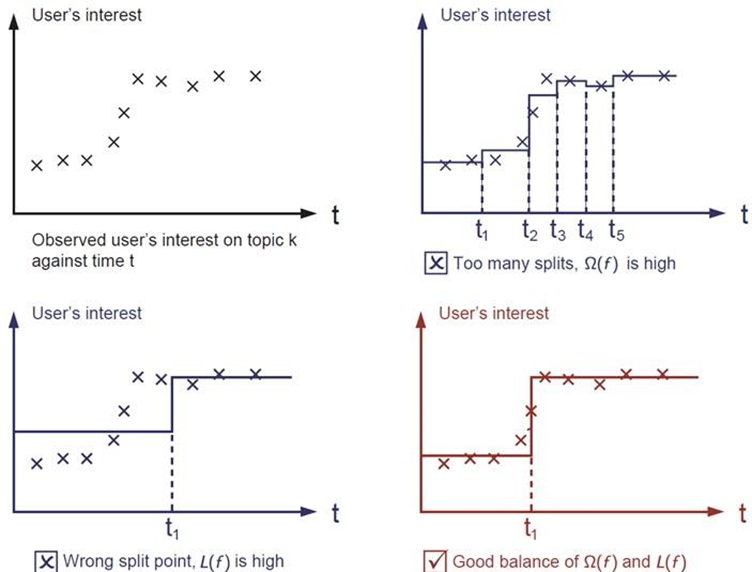图1，是真实的样本数据，要做的是找到拟合这些点的线。 图2，我们可以把这些点分成不同的区间段，区间段如果选的比较小，两个点一段，均值作为预测值。甚至还可以更小，小到一个点一段，类似于微分的思想。这样肯定会很好的拟合这些样本。 图3，如果选的区间段的跨度比较大，还以均值为预测值，会发现此时的误差会特别大。 上面说的L(f)是预测值与实际值之间的差值，是期望误差。Ω(f)表示方差误差，指复杂度。error = Ω(f) + L(f)，实际来讲不仅仅是降低期望误差，而是二者结合的一个最优。也就是图4的效果。 换句话说，图2是过拟合，图3是欠拟合，图4是比较理想的结果。
就像上图，现实中的样本数据往往都是非线性的，如果能直接找到曲线去拟合，那往往效果会更好。前两篇博客介绍的是线性回归，线性回归针对的是θ而言的，即

θ

j

θ_j

的最高次项是1次。对于样本本身而言，样本可以是非线性的也就是说最终得到的函数f:x->y；函数f(x)可以是非线性的，比如：曲线等。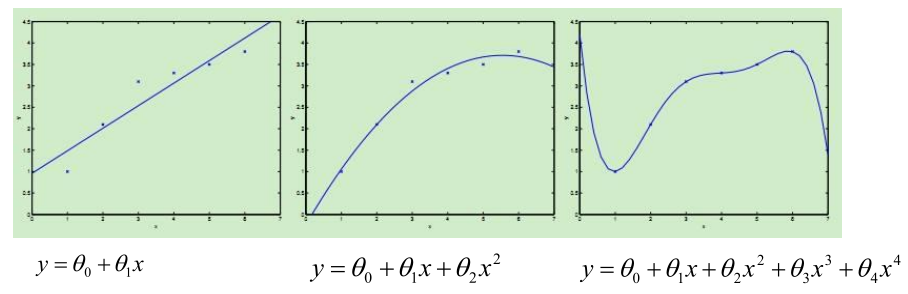很显然，如果用直线去拟合这种曲线的情况，结果会很不理想，后面的两张图的拟合效果好很多。这就是我们本文提到的多项式扩展。
代码
这里依然使用前面的“家庭用电预测”的样本数据，通过代码看一下时间与电压的多项式关系。
# 引入所需要的全部包
import sklearn
from sklearn.model_selection import train_test_split
from sklearn.linear_model import LinearRegression
from sklearn.preprocessing import StandardScaler
from sklearn.preprocessing import PolynomialFeatures
from sklearn.pipeline import Pipeline

import numpy as np
import matplotlib as mpl
import matplotlib.pyplot as plt
import pandas as pd
import time

# 创建一个时间字符串格式化字符串
def date_format(dt):
import time
t = time.strptime(' '.join(dt), '%d/%m/%Y %H:%M:%S')
return (t.tm_year, t.tm_mon, t.tm_mday, t.tm_hour, t.tm_min, t.tm_sec)

## 设置字符集，防止中文乱码
mpl.rcParams['font.sans-serif']=[u'simHei']
mpl.rcPar
ams['axes.unicode_minus']=False

# 加载数据
path = 'datas\household_power_consumption_200.txt' ## 200行数据
path = 'datas\household_power_consumption_1000.txt' ## 1000行数据

# 日期、时间、有功功率、无功功率、电压、电流、厨房用电功率、洗衣服用电功率、热水器用电功率
names2=df.columns
names=['Date', 'Time', 'Global_active_power', 'Global_reactive_power', 'Voltage', 'Global_intensity', 'Sub_metering_1', 'Sub_metering_2', 'Sub_metering_3']

# 异常数据处理(异常数据过滤)
new_df = df.replace('?', np.nan)
datas = new_df.dropna(axis=0,how = 'any') # 只要有数据为空，就进行删除操作

## 时间和电压之间的关系(Linear)
# 获取x和y变量, 并将时间转换为数值型连续变量
X = datas[names[0:2]]
X = X.apply(lambda x: pd.Series(date_format(x)), axis=1)
Y = datas[names].values

# 对数据集进行测试集合训练集划分
X_train,X_test,Y_train,Y_test = train_test_split(X, Y, test_size=0.2, random_state=0)

# 数据标准化
ss = StandardScaler()
X_train = ss.fit_transform(X_train) # 训练并转换
X_test = ss.transform(X_test) ## 直接使用在模型构建数据上进行一个数据标准化操作

# 模型训练
lr = LinearRegression()
lr.fit(X_train, Y_train) ## 训练模型

# 模型校验
y_predict = lr.predict(X_test) ## 预测结果

# 模型效果
print("准确率:",lr.score(X_test, Y_test))

## 预测值和实际值画图比较
t=np.arange(len(X_test))
plt.figure(facecolor='w')
plt.plot(t, Y_test, 'r-', linewidth=2, label=u'真实值')
plt.plot(t, y_predict, 'g-', linewidth=2, label=u'预测值')
plt.legend(loc = 'lower right')
plt.title(u"线性回归预测时间和功率之间的关系", fontsize=20)
plt.grid(b=True)#网格
plt.show()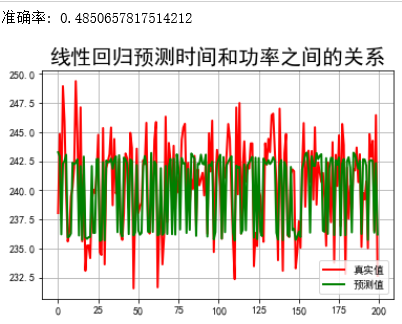发现：时间和功能之间的关系，不是那的契合，就是说找的这条直线没法很好拟合样本点的。那样本点是不是曲线形式，我们可不可以进行一下扩展？下面就采用了多项式扩展。 多项式扩展：将特征与特征之间进行整合，从而形成新的特征的一个过程；从数学空间上来讲，就是将低维度空间的点映射到高维度空间中。（比如说一维，平方后，就变成了二维。映射到高维之后，数据会更分散，一些特性就更容易体现出来）属于特征工程的一种操作。 作用：通过多项式扩展后，我们可以提高模型的准确率/效果。
## 时间和电压之间的关系(Linear-多项式)
# Pipeline：管道的意思，讲多个操作合并成为一个操作
# Pipleline总可以给定多个不同的操作，给定每个不同操作的名称即可，执行的时候，按照从前到后的顺序执行
# Pipleline对象在执行的过程中，当调用某个方法的时候，会调用对应过程的对应对象的对应方法
# eg：在下面这个案例中，调用了fit方法，
# 那么对数据调用第一步操作：PolynomialFeatures的fit_transform方法对数据进行转换并构建模型
# 然后对转换之后的数据调用第二步操作: LinearRegression的fit方法构建模型
# eg: 在下面这个案例中，调用了predict方法，
# 那么对数据调用第一步操作：PolynomialFeatures的transform方法对数据进行转换
# 然后对转换之后的数据调用第二步操作: LinearRegression的predict方法进行预测
models = [
Pipeline([
('Poly', PolynomialFeatures()), # 给定进行多项式扩展操作， 第一个操作：多项式扩展
('Linear', LinearRegression(fit_intercept=False)) # 第二个操作，线性回归
])
]

注：这里引入了Pipeline，管道的意思。之前每写都先fit，再transform。通过管道就可以把他们放到一起。
Poly表示名字，是自定义的，这一行属于第一步，也就是进行多项式扩展；Linear也是表示名字，是自定义，这一行属于第二步，也就是进行线性回归。
model = models
# 获取x和y变量, 并将时间转换为数值型连续变量
X = datas[names[0:2]]
X = X.apply(lambda x: pd.Series(date_format(x)), axis=1)
Y = datas[names]

# 对数据集进行测试集合训练集划分
X_train,X_test,Y_train,Y_test = train_test_split(X, Y, test_size=0.2, random_state=0)

# 数据标准化
ss = StandardScaler()
X_train = ss.fit_transform(X_train) # 训练并转换
X_test = ss.transform(X_test) ## 直接使用在模型构建数据上进行一个数据标准化操作

# 模型训练
t=np.arange(len(X_test))
N = 5
d_pool = np.arange(1,N,1) # 阶

注：阶，即表示扩展后的多项式的最高次
m = d_pool.size
clrs = [] # 颜色
for c in np.linspace(16711680, 255, m):
clrs.append('#%06x' % int(c))
line_width = 3

plt.figure(figsize=(12,6), facecolor='w')#创建一个绘图窗口，设置大小，设置颜色
#下面是对阶数的迭代
for i,d in enumerate(d_pool):
plt.subplot(N-1,1,i+1)
plt.plot(t, Y_test, 'r-', label=u'真实值', ms=10, zorder=N)
### 设置管道对象中的参数值，Poly是在管道对象中定义的操作名称， 后面跟参数名称；中间是两个下划线
model.set_params(Poly__degree=d) ## 设置多项式的阶乘
model.fit(X_train, Y_train) # 模型训练
# Linear是管道中定义的操作名称
# 获取线性回归算法模型对象
lin = model.get_params()['Linear']
output = u'%d阶，系数为：' % d
# 判断lin对象中是否有对应的属性
if hasattr(lin, 'alpha_'):
idx = output.find(u'系数')
output = output[:idx] + (u'alpha=%.6f, ' % lin.alpha_) + output[idx:]
if hasattr(lin, 'l1_ratio_'):
idx = output.find(u'系数')
output = output[:idx] + (u'l1_ratio=%.6f, ' % lin.l1_ratio_) + output[idx:]
print (output, lin.coef_.ravel())

# 模型结果预测
y_hat = model.predict(X_test)
# 计算评估值
s = model.score(X_test, Y_test)

# 画图
z = N - 1 if (d == 2) else 0
label = u'%d阶, 准确率=%.3f' % (d,s)
plt.plot(t, y_hat, color=clrs[i], lw=line_width, alpha=0.75, label=label, zorder=z)
plt.legend(loc = 'upper left')
plt.grid(True)
plt.ylabel(u'%d阶结果' % d, fontsize=12)

## 预测值和实际值画图比较
plt.suptitle(u"线性回归预测时间和功率之间的多项式关系", fontsize=20)
plt.grid(b=True)
plt.show()

看一下输出的参数： 1阶，系数为： [2.39926650e+02 0.00000000e+00 0.00000000e+00 3.97781449e+00 8.73334650e-01 1.70647992e-01 0.00000000e+00] 2阶，系数为： [ 1.23998300e+02 3.55271368e-14 -7.81597009e-14 5.34497071e+01 2.95068077e+00 2.69407641e-01 -5.32907052e-15 -3.55271368e-15 8.88178420e-16 0.00000000e+00 0.00000000e+00 0.00000000e+00 0.00000000e+00 0.00000000e+00 0.00000000e+00 0.00000000e+00 0.00000000e+00 0.00000000e+00 1.02461452e+02 -2.50100464e+01 -5.18469319e-01 0.00000000e+00 -1.02427364e+01 -4.65982219e-01 0.00000000e+00 -3.55472266e-02 0.00000000e+00 .00000000e+00] 3阶，系数为： [ 1.06303324e+12 -7.52773669e+11 2.12816760e+12 -9.53433863e+12 1.50224363e+11 1.24753680e+11 -2.10445177e+11 -2.86373371e+11 -2.73949767e+11 1.63670539e+11 5.97002023e+10 -2.79408605e+11 -3.55726203e+11 -2.59005902e+11 6.79712021e+10 -1.44760428e+10 5.20112328e+10 -9.76562500e-04 2.60610968e+12 -6.05309076e+10 -5.02678348e+10 0.00000000e+00 1.10827637e+00 3.45336914e-01 0.00000000e+00 5.85937500e-03 0.00000000e+00 0.00000000e+00 0.00000000e+00 0.00000000e+00 0.00000000e+00 0.00000000e+00 0.00000000e+00 0.00000000e+00 0.00000000e+00 0.00000000e+00 0.00000000e+00 0.00000000e+00 0.00000000e+00 0.00000000e+00 0.00000000e+00 0.00000000e+00 0.00000000e+00 0.00000000e+00 0.00000000e+00 0.00000000e+00 0.00000000e+00 0.00000000e+00 0.00000000e+00 0.00000000e+00 0.00000000e+00 0.00000000e+00 0.00000000e+00 0.00000000e+00 0.00000000e+00 0.00000000e+00 0.00000000e+00 0.00000000e+00 0.00000000e+00 0.00000000e+00 0.00000000e+00 0.00000000e+00 0.00000000e+00 0.00000000e+00 9.10600353e+12 -1.50224363e+11 -1.24753680e+11 0.00000000e+00 -6.41564941e+00 -6.71470642e-01 0.00000000e+00 -1.84478760e-01 0.00000000e+00 0.00000000e+00 4.48028564e+00 2.57629395e-01 0.00000000e+00 -2.65136719e-01 0.00000000e+00 0.00000000e+00 -2.44018555e-01 0.00000000e+00 0.00000000e+00 0.00000000e+00] 4阶，系数为： [ 1.98236950e+13 -6.36266368e+12 -8.19197778e+11 -2.41835460e+13 2.40054791e+12 1.10773332e+12 2.52245897e+12 2.26093380e+12 -4.64598235e+11 -3.10328767e+11 6.98913673e+11 9.69576533e+11 3.36682879e+11 5.31213939e+11 -1.93230766e+11 -7.71628230e+11 3.76054179e+11 1.38354357e+11 5.03666951e+12 -1.14372116e+13 -6.42706951e+11 -1.04958920e+11 9.69966926e+12 2.54696605e+11 -1.21838302e+11 5.77387250e+12 4.40390380e+10 -1.45775272e+10 -1.40971612e+10 -5.91093717e+08 2.89672374e+07 5.29743161e+06 -7.99508243e+05 0.00000000e+00 0.00000000e+00 0.00000000e+00 0.00000000e+00 0.00000000e+00 0.00000000e+00 0.00000000e+00 0.00000000e+00 0.00000000e+00 0.00000000e+00 0.00000000e+00 0.00000000e+00 0.00000000e+00 0.00000000e+00 0.00000000e+00 0.00000000e+00 0.00000000e+00 0.00000000e+00 0.00000000e+00 0.00000000e+00 0.00000000e+00 0.00000000e+00 0.00000000e+00 0.00000000e+00 0.00000000e+00 0.00000000e+00 0.00000000e+00 0.00000000e+00 0.00000000e+00 0.00000000e+00 0.00000000e+00 8.80822476e+12 1.81817615e+12 -1.02861244e+12 0.00000000e+00 -3.90835262e+12 -1.02626607e+11 0.00000000e+00 -2.32650507e+12 0.00000000e+00 0.00000000e+00 -1.53422852e+01 -2.18774414e+00 0.00000000e+00 -5.88867188e-01 0.00000000e+00 0.00000000e+00 -2.44140625e-01 0.00000000e+00 0.00000000e+00 0.00000000e+00 0.00000000e+00 0.00000000e+00 0.00000000e+00 0.00000000e+00 0.00000000e+00 0.00000000e+00 0.00000000e+00 0.00000000e+00 0.00000000e+00 0.00000000e+00 0.00000000e+00 0.00000000e+00 0.00000000e+00 0.00000000e+00 0.00000000e+00 0.00000000e+00 0.00000000e+00 0.00000000e+00 0.00000000e+00 0.00000000e+00 0.00000000e+00 0.00000000e+00 0.00000000e+00 0.00000000e+00 0.00000000e+00 0.00000000e+00 0.00000000e+00 0.00000000e+00 0.00000000e+00 0.00000000e+00 0.00000000e+00 0.00000000e+00 0.00000000e+00 0.00000000e+00 0.00000000e+00 0.00000000e+00 0.00000000e+00 0.00000000e+00 0.00000000e+00 0.00000000e+00 0.00000000e+00 0.00000000e+00 0.00000000e+00 0.00000000e+00 0.00000000e+00 0.00000000e+00 0.00000000e+00 0.00000000e+00 0.00000000e+00 0.00000000e+00 0.00000000e+00 0.00000000e+00 0.00000000e+00 0.00000000e+00 0.00000000e+00 0.00000000e+00 0.00000000e+00 0.00000000e+00 0.00000000e+00 0.00000000e+00 0.00000000e+00 0.00000000e+00 0.00000000e+00 0.00000000e+00 0.00000000e+00 0.00000000e+00 0.00000000e+00 0.00000000e+00 0.00000000e+00 0.00000000e+00 0.00000000e+00 0.00000000e+00 0.00000000e+00 0.00000000e+00 0.00000000e+00 0.00000000e+00 0.00000000e+00 0.00000000e+00 0.00000000e+00 0.00000000e+00 0.00000000e+00 0.00000000e+00 0.00000000e+00 0.00000000e+00 0.00000000e+00 0.00000000e+00 0.00000000e+00 0.00000000e+00 0.00000000e+00 0.00000000e+00 0.00000000e+00 -1.83344615e+13 1.04699427e+13 1.96360556e+11 0.00000000e+00 -9.69966926e+12 -2.54696605e+11 0.00000000e+00 -5.77387250e+12 0.00000000e+00 0.00000000e+00 1.53059082e+01 -6.72119141e+00 0.00000000e+00 -2.19726562e-01 0.00000000e+00 0.00000000e+00 3.61328125e-02 0.00000000e+00 0.00000000e+00 0.00000000e+00 -3.05761719e+00 -3.54296875e+00 0.00000000e+00 -4.14062500e-01 0.00000000e+00 0.00000000e+00 2.08007812e-01 0.00000000e+00 0.00000000e+00 0.00000000e+00 -2.53906250e-02 0.00000000e+00 0.00000000e+00 0.00000000e+00 0.00000000e+00]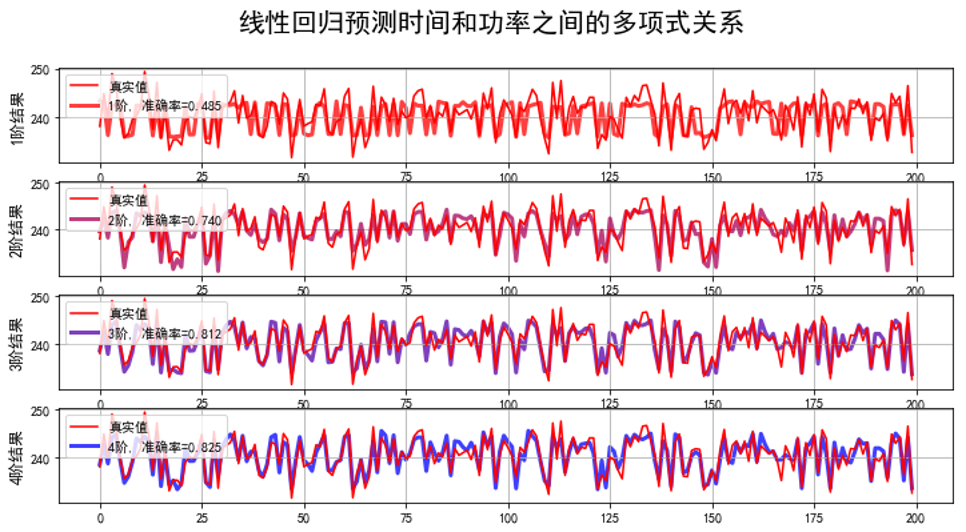发现：随着阶数增加，准确率在提高。通过多项式扩展能进行很好的拟合
分析
再结合下面一张简化的图，来看一下数据更深层的内容。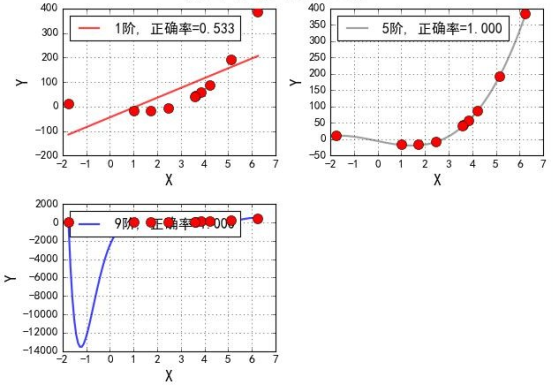对应的系数：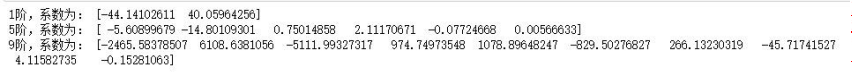图中红色的点是样本点，如果用线来拟合的话，近似一条二次函数曲线，而做线性回归，找的是直线，直线和实际分布就不太拟合。通过提高阶数，能对数据进行很好的拟合（图2），但当阶数特别大时（图3），出现了很大的拐线。其实这是出现了严重的过拟合。 过拟合：如果模型在训练集上效果非常好，而在测试集上效果不好，那么认为这个时候存在过拟合的情况，多项式扩展的时候，如果指定的阶数比较大，那么有可能导致过拟合。从线性回归模型中来讲，我们认为训练出来的模型参数值越大，就表示越存在过拟合的情况。（对于过拟合的情况该如何解决，最常用的就是正则化，也就是加入惩罚项，详情内容，下篇博客会介绍） 也就是越复杂，越会出现过拟合。
对于线性回归，可能就是参数过多（阶数过大、参数值比较大）对于决策树，就是叶子节点过多…
换一个角度来说： 在线性回归中，我们可以通过多项式扩展将低维度的数据扩展成为高维度的数据，从而可以使用线性回归模型来解决问题。也就是说对于二维空间中不是线性可分的数据，将其映射到高维空间中后，变成了线性可分的数据。 两维线性模型:

h

θ

(

x

1

,

x

2

)

=

θ

0

+

θ

1

x

1

+

θ

2

x

2

(

x

1

,

x

2

)

多

项

式

扩

展

→

(

x

1

,

x

2

,

x

1

2

,

x

2

2

,

x

1

x

2

)

h_θ (x_1,x_2 )=θ_0+θ_1 x_1+θ_2 x_2 \\ (x_1,x_2 ) \underrightarrow{多项式扩展} (x_1,x_2,x_1^2,x_2^2,x_1 x_2)

五维线性模型:

h

θ

(

x

1

,

x

2

)

=

θ

0

+

θ

1

x

1

+

θ

2

x

2

+

θ

3

x

1

2

+

θ

4

x

2

2

+

θ

5

x

1

x

2

等

价

→

h

θ

(

x

1

,

x

2

)

=

θ

0

+

θ

1

z

1

+

θ

2

z

2

+

θ

3

z

3

+

θ

4

z

4

+

θ

5

z

5

h_θ (x_1,x_2 )=θ_0+θ_1 x_1+θ_2 x_2+θ_3 x_1^2+θ_4 x_2^2+θ_5 x_1 x_2 \\ \underrightarrow{等价} h_θ (x_1,x_2 )=θ_0+θ_1 z_1+θ_2 z_2+θ_3 z_3+θ_4 z_4+θ_5 z_5

通过等价变化后，可以看出，从本质上讲，多项式拟合也是一个线性模型。经过上面的分析可以知道，多项式拟合其实是两个过程：
对原始特征向量 x 做多项式特征生成，得到新的特征z对新的特征 z 做线性回归
其实多项式扩展的这种方法稍想一下就会发现，除了可能的过拟合的问题之外还有： 在最初的例子里做了一个二阶多项式的转换，对一个二维空间做映射，选择的新空间是原始空间的所有一阶和二阶的组合，得到了5个维度；如果原始空间是三维，那么我们会得到9维的新空间；如果原始空间是n维，那么我们会得到一个n(n+3)/2 维的新空间；这个数目是呈爆炸性增长的，这给计算带来了非常大的困难。（SVM对于非线性可分就是用的升维的方式，只不过采用的是更有效的核函数）
总结
事实上，线性回归就是特殊的多项式回归，这很好理解，最后，我想说的是，不考虑过拟合之类的问题，理论上来说，多项式回归应该是可以拟合任何的数据的，因为我们有泰勒定理，任意的函数都可以通过泰勒级数逼近，或许我们构建的多项式过于复杂，有很多项是不需要的（因为多项式回归必须把同一次项的所有情况都要考虑，比如两个特征的三次多项式，只要是特征的幂是三就需要称为多项式的项，而不仅仅是每个特征的一二三次幂），但是我们也要知道，在训练模型的过程中，对数据影响不大的项的参数是会最终趋于零的，所以不考虑计算量、过拟合等问题，哪怕我们只用多项式回归这个方法，也足够拟合任意的数据集了。
展开全文• 线性回归(Linear Regression) 主要分析两种线性回归模型： 简单线性回归(Simple Linear Regression): 只有两类输出(e.g. (0,1)) 多元线性回归(Multiple Linear Regression): 有多个分类输出(e.g. (0,1,2,3)) ...
线性回归(Linear Regression)
回归问题与分类问题的区别： 回归是预测连续的值，分类是预测离散的值。
主要分析两种线性回归模型：
简单线性回归(Simple Linear Regression): 只有两类输出(e.g. (0,1))多元线性回归(Multiple Linear Regression): 有多个分类输出(e.g. (0,1,2,3))
Simple Linear Regression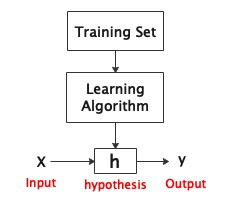对于简单线性回归，h的假设函数

h

θ

(

x

)

=

θ

0

+

θ

1

x

h_\theta(x)=\theta_0+\theta_1x

Cost Function (误差)

J

(

θ

0

,

θ

1

)

=

1

2

m

∑

i

=

1

m

(

h

θ

(

x

(

i

)

)

−

y

(

i

)

)

2

J(\theta_0,\theta_1)=\frac{1}{2m}\sum_{i=1}^{m}(h_\theta(x^{(i)})-y^{(i)})^2

cost function为假设函数

h

θ

(

x

)

h_\theta(x)

的误差和，目标是使该误差最小化，即对于

(

θ

0

,

θ

1

)

(\theta_0,\theta_1)

使得

m

i

n

i

m

i

z

e

J

(

θ

0

,

θ

1

)

minimize J(\theta_0,\theta_1)

成立。其中

m

m

为训练样本数量。
Optimisation(优化)
选用优化算法来取得最好的预测效果，即将代价函数

J

(

θ

0

,

θ

1

)

J(\theta_0,\theta_1)

最小化。

θ

j

:

=

θ

j

−

α

∂

∂

θ

j

J

(

θ

0

,

θ

1

)

\theta_j:=\theta_j-\alpha\frac{\partial}{\partial\theta_j}J(\theta_0,\theta_1)

α

\alpha

为学习率(learning rare)，即步长。且

θ

0

,

θ

1

\theta_0,\theta_1

同时更新：

t

e

m

p

0

:

=

θ

0

−

α

∂

∂

θ

0

J

(

θ

0

,

θ

1

)

temp0:=\theta_0-\alpha\frac{\partial}{\partial\theta_0}J(\theta_0,\theta_1)

t

e

m

p

1

:

=

θ

1

−

α

∂

∂

θ

1

J

(

θ

0

,

θ

1

)

temp1:=\theta_1-\alpha\frac{\partial}{\partial\theta_1}J(\theta_0,\theta_1)

θ

0

:

=

t

e

m

p

0

\theta_0:=temp0

θ

1

:

=

t

e

m

p

1

\theta_1:=temp1

将梯度下降算法应用到cost function:

t

h

e

t

a

0

:

=

θ

0

−

α

1

m

∑

i

=

1

m

(

h

θ

(

x

(

i

)

)

−

y

(

i

)

)

theta_0:=\theta_0-\alpha\frac{1}{m}\sum_{i=1}^{m}(h_\theta(x^{(i)})-y^{(i)})

t

h

e

t

a

1

:

=

θ

1

−

α

1

m

∑

i

=

1

m

(

h

θ

(

x

(

i

)

)

−

y

(

i

)

)

∗

x

(

i

)

theta_1:=\theta_1-\alpha\frac{1}{m}\sum_{i=1}^{m}(h_\theta(x^{(i)})-y^{(i)})*x^(i)

此时获得局部最优解，又因为三维函数

J

(

θ

0

,

θ

1

)

−

θ

0

−

θ

1

J(\theta_0,\theta_1)-\theta_0-\theta_1

为突函数(碗状)，因此局部最优解即为全局最优解，即得到假设函数

h

θ

(

x

)

h_\theta(x)

的最优解。
Multiple Linear Regression
Cost Function

J

(

θ

)

=

1

2

m

∑

i

=

1

m

(

h

θ

(

x

(

i

)

)

−

y

(

i

)

)

2

J(\theta)=\frac{1}{2m}\sum_{i=1}^{m}(h_\theta(x^{(i)})-y^{(i)})^2

Where

θ

=

θ

0

,

θ

1

,

θ

2

.

.

.

.

.

.

θ

n

\theta=\theta_0,\theta_1,\theta_2......\theta_n

Thus, 多元线性回归的假设函数为:

h

θ

(

x

)

=

θ

T

x

=

θ

0

x

0

+

θ

1

x

1

+

.

.

.

.

.

.

+

θ

n

x

n

h_\theta(x)=\theta^{T}x=\theta_0x_0+\theta_1x_1+......+\theta_nx_n

多元线性回归的梯度下降计算方式为：

θ

j

:

=

θ

j

−

α

∂

∂

θ

j

J

(

θ

)

\theta_j:=\theta_j-\alpha\frac{\partial}{\partial\theta_j}J(\theta)

=

>

θ

j

:

=

θ

j

−

α

1

m

∑

i

=

1

m

(

h

θ

(

x

(

i

)

)

−

y

(

i

)

)

∗

x

j

(

i

)

=>\theta_j:=\theta_j-\alpha\frac{1}{m}\sum_{i=1}^{m}(h_\theta(x^{(i)})-y^{(i)})*x^{(i)}_j

Where

j

=

0

,

1

,

2......

n

j=0,1,2......n

多项式回归
假设函数：

h

θ

(

x

)

=

θ

T

x

=

θ

0

+

θ

1

x

+

θ

2

x

2

+

.

.

.

.

.

.

+

θ

n

x

n

h_\theta(x)=\theta^{T}x=\theta_0+\theta_1x+\theta_2x^2+......+\theta_nx^n

正规方程(Normal Equation)

θ

=

(

X

T

X

)

−

1

X

T

y

\theta=(X^TX)^{-1}X^Ty

=

>

m

i

n

θ

J

(

θ

)

=>min_{\theta}J(\theta)

用正规方程进行优化算法的实现。
梯度下降与正规方程的区别

α

\alpha

No need to choose

α

\alpha

Need many iterationsDon’t need iterationWorks well even n(特征数量) is largeNeed to compute

(

X

T

X

)

−

1

(X^TX)^{-1}

(需要O(n^3)时间复杂度)–Slow if n(特征数量) is very large
展开全文机器学习
• 同样，线性回归模型一经训练（不论是标准方程还是其他算法），预测就非常快速：因为计算复杂度相对于想要预测的实例数量特征数量来说，都是线性的。换句话说，对两倍的实例（或者是两倍的特征数）进行预测，大概...


文章目录
一、线性回归1.线性模型2.训练线性回归模型3.标准方程4.标准方程的局限性5.梯度下降算法5.1批量梯度下降5.2随机梯度下降5.3小批量梯度下降

二、多项式回归1.多项式回归模型2.训练多项式回归模型3.学习曲线4.偏差/方差权衡

一、线性回归
1.线性模型
概括地说，线性模型就是对输入特征加权求和，再加上一个偏置项（也称为截距项）的常数，以此进行预测。 线性回归模型预测公式：

y

^

=

θ

0

+

θ

1

x

1

+

θ

2

x

2

+

⋯

+

θ

n

x

n

\hat{y}=\theta_0+\theta_1x_1+\theta_2x_2+\cdots+\theta_nx_n

y

^

\hat{y}

是预测值n是特征的数量

x

i

x_i

是第

i

i

个特征值

θ

j

\theta_j

是第

j

j

个模型参数（包括偏置项

θ

0

\theta_0

以及特征权重

θ

1

,

θ

2

,

⋯

,

θ

n

\theta_1,\theta_2,\cdots,\theta_n

）
这可以用更为简洁的向量化形式表达：

y

^

=

h

θ

(

X

)

=

θ

T

⋅

X

\hat{y}=h_\theta(X)=\theta^T\cdot X

θ

\theta

是模型的参数向量，包括偏置项

θ

0

\theta_0

以及特征权重

θ

1

\theta_1

到

θ

n

\theta_n

θ

T

\theta^T

是

θ

\theta

的转置向量（为行向量，而不再是列向量）

X

X

是实例的特征向量，包括从

x

0

x_0

到

x

n

x_n

，

x

0

x_0

永远为1

θ

T

⋅

X

\theta^T\cdot X

是

θ

T

\theta^T

和

X

X

的点积

h

θ

h_\theta

是使用模型参数

θ

\theta

的假设函数
2.训练线性回归模型
训练模型就是设置模型参数直到模型最适应训练集的过程。要达到这个目的，我们首先需要知道怎么衡量模型对训练数据的拟合程度是好是差。回归模型最常见的性能指标是均方根误差（RMSE）。因此，在训练线性回归模型时，我们需要找到最小化RMSE的

θ

\theta

值。在实践中，将均方误差（MSE）最小化比最小化RMSE更为简单，二者效果相同（因为使函数最小化的值，同样也使其平方根最小）。
线性回归模型的MSE成本函数：

M

S

E

(

X

,

h

θ

)

=

1

m

∑

i

=

1

m

(

θ

T

⋅

X

(

i

)

−

y

(

i

)

)

2

MSE(X,h_\theta)=\frac1m\sum^m_{i=1}(\theta^T\cdot X^{(i)}-y^{(i)})^2

m

m

是所使用的数据集中实例的数量

X

(

i

)

X^{(i)}

是数据集中，第

i

i

个实例的所有特征值的向量（标签特征除外），

y

(

i

)

y^{(i)}

是标签（也就是我们期待该实例的输出值）

X

X

是数据集中所有实例的所有特征值的矩阵（标记特征除外）。每个实例为一行。

h

θ

h_\theta

是系统的预测函数，也称为一个假设

M

S

E

(

X

,

h

θ

)

MSE(X,h_\theta)

是使用假设

h

θ

h_\theta

在实例上测量的成本函数。
3.标准方程
为了得到使成本函数最小的

θ

\theta

值，有一个闭式解方法——也就是一个直接得出结果的数学方程，即标准方程：

θ

^

=

(

X

T

⋅

X

)

−

1

⋅

X

T

⋅

y

\hat{\theta}=(X^T\cdot X)^{-1}\cdot X^T\cdot y

θ

^

\hat{\theta}

是使成本函数最小的

θ

\theta

值。

y

y

是包含

y

(

1

)

y^{(1)}

到

y

(

m

)

y^{(m)}

的目标值向量。
我们生成一些线性数据来测试这个公式：
import numpy as np
X=2*np.random.rand(100,1)
y=4+3*X+np.random.randn(100,1)
plt.plot(X,y,'b.')
plt.axis([0,2,0,15])
plt.show()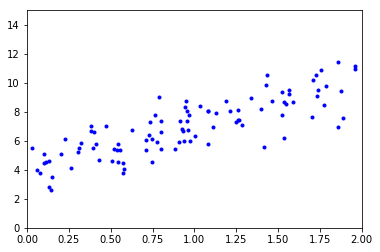使用标准方程来计算

θ

^

\hat{\theta}

。使用NumPy的线性代数模块（np.linalg）中的inv()函数来对矩阵求逆，并用dot()方法计算矩阵的内积：
X_b=np.c_[np.ones((100,1)),X] #按行连接两个矩阵
theta_best=np.linalg.inv(X_b.T.dot(X_b)).dot(X_b.T).dot(y)

我们实际用来生成数据的函数是

y

=

4

+

3

x

0

+

高

斯

噪

声

y=4+3x_0+高斯噪声

。来看看公式的结果：
theta_best

array([[ 4.0929349 ],
[ 3.00811997]])

我们期待的是

θ

0

=

4

,

θ

1

=

3

\theta_0=4,\theta_1=3

得到的是

θ

0

=

4.093

,

θ

1

=

3.008

\theta_0=4.093,\theta_1=3.008

。非常接近，噪声的存在使其不可能完全还原为原本的函数。
现在可以用

θ

^

\hat{\theta}

做出预测：
X_new=np.array([,])
X_new_b=np.c_[np.ones((2,1)),X_new]
y_predict=X_new_b.dot(theta_best)
y_predict

array([[  4.0929349 ],
[ 10.10917484]])

绘制模型的预测结果：
plt.plot(X,y,'b.')
plt.plot(X_new,y_predict,'r-')
plt.axis([0,2,0,15])
plt.show()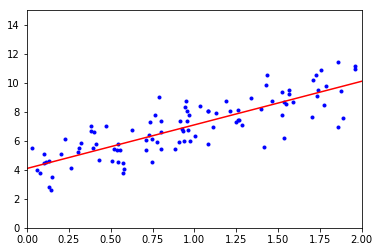Scikit-Learn的等效代码如下所示：
from sklearn.linear_model import LinearRegression
lin_reg=LinearRegression()
lin_reg.fit(X,y)

LinearRegression(copy_X=True, fit_intercept=True, n_jobs=1, normalize=False)

Scikit-Learn将偏置项（intercept_）和特征权重（coef_）分离开：
lin_reg.intercept_,lin_reg.coef_

(array([ 4.0929349]), array([[ 3.00811997]]))

预测结果也是一样的：
X_new=np.array([,])
y_predict=lin_reg.predict(X_new)
y_predict

 array([[  4.0929349 ],
[ 10.10917484]])

4.标准方程的局限性
标准方程求逆的矩阵

X

T

⋅

X

X^T\cdot X

，是一个nXn矩阵（n是特征数量）。对这种矩阵求逆的计算复杂度通常为

O

(

n

2.4

)

O(n^{2.4})

到

O

(

n

3

)

O(n^3)

之间（取决于计算实现）。换句话说，如果将特征数量翻倍，那么计算时间将乘以大约

2

2.4

=

5.3

2^{2.4}=5.3

倍到

2

3

=

8

2^3=8

倍之间。 特征数量比较大（例如100000）时，标准方程的计算将极其缓慢。
好的一面是，相对于训练集中的实例数量（

O

(

m

)

O(m)

）来说，方程是线性的，所以能够有效地处理大量的训练集，只要内存足够。
同样，线性回归模型一经训练（不论是标准方程还是其他算法），预测就非常快速：因为计算复杂度相对于想要预测的实例数量和特征数量来说，都是线性的。换句话说，对两倍的实例（或者是两倍的特征数）进行预测，大概需要两倍的时间。
5.梯度下降算法
梯度下降是一种非常通用的优化算法，能够为大范围的问题找到最优解。梯度下降的中心思想就是迭代地调整参数从而使成本函数最小化：通过测量参数向量

θ

\theta

相关的误差函数的局部梯度，并不断沿着降低梯度的方向调整，直到梯度降为0，到达最小值！
具体来说，首先使用一个随机的

θ

\theta

值（这被称为随机初始化），然后逐步改进，每次踏出一步，每一步都尝试降低一点成本函数（如MSE），直到算法收敛出一个最小值。
梯度下降中一个重要参数是每一步的步长，这取决于超参数学习率。如果学习率太低，算法需要经过大量迭代才能收敛，这将耗费很长时间。反之，如果学习率太高，会导致算法发散，值越来越大，最后无法找到好的解决方案。
梯度下降的两个主要挑战是：如果随机初始化不好，一种可能是算法会收敛到一个局部最小值，而不是全局最小值。另一种可能是算法需要经过很长时间的迭代过程，如果我们停下得太早，将永远达不到全局最小值。
幸好，线性回归模型的MSE成本函数恰好是个凸函数，这意味着连接曲线上任意两个点的线段永远不会跟曲线相交。也就是说不存在局部最小，只有一个全局最小值。它同时也是一个连续函数，所以斜率不会产生陡峭的变化。这两件事保证的结论是：即便是乱走，梯度下降都可以趋近到全局最小值（只要等待时间足够长，学习率也不是太高）。
应用梯度下降时，需要保证所有特征值的大小比例都差不多，否则收敛的时间会长很多。
5.1批量梯度下降
要实现梯度下降，我们需要计算每个模型关于参数

θ

j

\theta_j

的成本函数的梯度。换言之，我们需要计算的是如果改变

θ

j

\theta_j

，成本函数会改变多少。这被称为偏导数。
关于参数

θ

j

\theta_j

的成本函数的偏导数：

δ

δ

θ

j

M

S

E

(

θ

)

=

2

m

∑

i

=

1

m

(

θ

T

⋅

X

(

i

)

−

y

(

i

)

)

x

j

(

i

)

\frac{\delta}{\delta\theta_j}MSE(\theta)=\frac2m\sum^m_{i=1}(\theta^T\cdot X^{(i)}-y^{(i)})x_j^{(i)}

如果不想单独计算这些梯度，可以使用成本函数的梯度向量计算公式对其进行一次性计算。梯度向量，记作

∇

θ

M

S

E

(

θ

)

\nabla_\theta MSE(\theta)

，包含所有成本函数（每个模型参数一个）的偏导数：

∇

θ

M

S

E

(

θ

)

=

2

m

X

T

⋅

(

X

⋅

θ

−

y

)

\nabla_\theta MSE(\theta)=\frac2mX^T\cdot (X\cdot \theta-y)

公式在计算梯度下降每一步时，都是基于完整的训练集X。这就是为什么该算法会被称为批量梯度下降：每一步都使用整批训练数据。因此，面对非常庞大的训练集时，算法会变得极慢。但是，梯度下降算法随特征数量扩展的表现比较好：如果要训练的线性模型拥有几十万个特征，使用梯度下降比标准方程要快得多。
一旦有了梯度向量，用梯度向量乘以学习率

η

\eta

就可以确定下坡步长的大小：

θ

(

n

e

x

t

s

t

e

p

)

=

θ

−

η

∇

θ

M

S

E

(

θ

)

\theta^{(next\;step)}=\theta-\eta\nabla_\theta MSE(\theta)

批量梯度下降算法的快速实现：
eta=0.1 #学习率
n_iterations=1000 #迭代次数
m=100
theta=np.random.randn(2,1) #随机初始化
for iteration in range(n_iterations):

代码不是太难，看看产生的结果theta:
theta

array([[ 4.0929349 ],
[ 3.00811997]])

与标准方程的结果完全一致，梯度下降表现完美。但如果使用了其他的学习率eta，算法可能不会表现得这么完美。要找到合适的学习率，可以使用网格搜索（GridSearchCV）。但是我们可能需要限制迭代次数，这样网格搜索可以淘汰掉那些收敛耗时太长的模型。
要怎么限制迭代次数呢？如果设置太低，算法可能在离最优解还很远时就停了；但是如果设置得太高，模型达到最优解后，继续迭代参数不再变化，又会浪费时间。一个简单的办法是，在开始时设置一个非常大的迭代次数，但是当梯度向量的值变得很微小时中断算法——也就是当它的范数变得低于

ε

\varepsilon

（容差）时，因为这时梯度下降已经几乎到达了最小值。
5.2随机梯度下降
批量梯度下降的主要问题是它要用整个训练集来计算每一步的梯度，所以训练集很大时，算法会特别慢。与之相反的极端是随机梯度下降，每一步在训练集中随机选择一个实例，并且仅基于该单个实例来计算梯度。显然，这让算法变得快多了，因为每个迭代都只需要操作少量的数据。它也可以被用来训练海量的数据集，因为每次迭代只需要在内存中运行一个实例即可。
另一方面，由于算法的随机性质，它比批量梯度下降要不规则得多。成本函数将不再是缓慢降低直到抵达最小值，而是不断上上下下，但是从整体来看，还是在慢慢下降。随着时间推移，最终会非常接近最小值，但是即使它到达了最小值，依旧还会持续反弹，永远不会停止。所以算法停下来的参数值肯定是足够好的，但不是最优的。
随机性的好处在于可以逃离局部最优，但缺点是永远定位不出最小值。要解决这个困境，有一个办法是逐步降低学习率。开始的步长比较大（这有助于快速进展和逃离局部最小值），然后越来越小，让算法尽量靠近全局最小值。这个过程叫作模拟退火，因为它类似于冶金时熔化的金属慢慢冷却的退火过程。确定每个迭代学习率的函数叫作学习计划。如果学习率降得太快，可能会陷入局部最小值，甚至是停留在走向最小值的半途中。如果学习率降得太慢，则需要太长时间才能跳到差不多最小值附近，如果提早结束训练，可能只得到一个次优的解决方案。
下面这段代码使用了一个简单的学习计划实现随机梯度下降：
n_epochs=50
t0,t1=5,50 #学习计划超参数
def learning_schedule(t): #学习计划函数
return t0/(t+t1)
theta=np.random.randn(2,1) #随机初始化
for epoch in range(n_epochs):
for i in range(m):
random_index=np.random.randint(m) #随机选择一个实例
xi=X_b[random_index:random_index+1]
yi=y[random_index:random_index+1]
eta=learning_schedule(epoch*m+i)

前面的批量梯度下降需要在整个训练集上迭代1000次，而这段代码只迭代了50次就得到了一个相当不错的解：
theta

array([[ 4.07034981],
[ 3.01321888]])

训练过程的前10步（虚线表示起点）：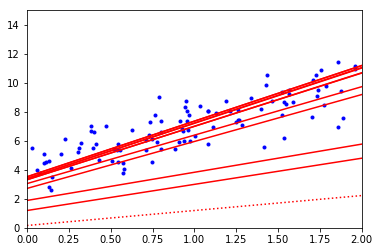在Scikit-Learn里，用SGD执行线性回归可以使用SGDRegression类，其默认优化的成本函数是平方误差。下面这段代码从学习率0.1开始（eta0=0.1），使用默认的学习计划（跟前面的学习计划不同）运行了50轮，而且没有使用任何正则化（penalty=None）：
from sklearn.linear_model import SGDRegressor
sgd_reg=SGDRegressor(n_iter=50,penalty=None,eta0=0.1)
sgd_reg.fit(X,y.ravel())

SGDRegressor(alpha=0.0001, average=False, epsilon=0.1, eta0=0.1,
fit_intercept=True, l1_ratio=0.15, learning_rate='invscaling',
loss='squared_loss', max_iter=None, n_iter=50, penalty=None,
power_t=0.25, random_state=None, shuffle=True, tol=None, verbose=0,
warm_start=False)

我们再次得到了一个跟标准方程的解非常相近的解决方案：
sgd_reg.intercept_,sgd_reg.coef_

(array([ 4.07553058]), array([ 3.00980982]))

5.3小批量梯度下降
一旦理解了批量梯度下降和随机梯度下降，小批量梯度下降算法就非常容易理解了：每一步的梯度计算，既不是基于整个训练集（如批量梯度下降）也不是基于单个实例（如随机梯度下降），而是基于一小部分随机的实例集也就是小批量。相比随机梯度下降，小批量梯度下降的主要优势在于可以从矩阵运算的硬件优化中获得显著的性能提升，特别是需要用到图形处理器时。
这个算法在参数空间层面的前进过程也不像SGD那样不稳定，特别是批量较大时。所以小批量梯度下降最终会比SGD更接近最小值一些。但是另一方面，它可能更难从局部最小值中逃脱。
二、多项式回归
1.多项式回归模型
如果数据比简单的直线更为复杂，该怎么办？令人意想不到的是，其实我们也可以用线性模型来拟合非线性数据。一个简单的方法就是将每个特征的幂次方添加为一个新特征，然后在这个拓展过的特征集上训练线性模型。这种方法被称为多项式回归。
2.训练多项式回归模型
首先，基于简单的二次方程制造一些非线性数据（添加随机噪声）：
m=100
X=6*np.random.rand(m,1)-3
y=0.5*X**2+X+2+np.random.randn(m,1)
plt.plot(X,y,'m.')
plt.axis([-3,3,0,10])
plt.show()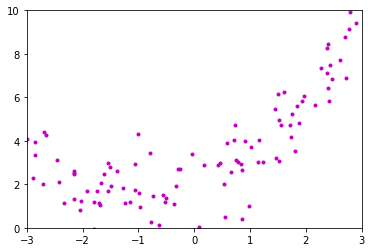显然，直线永远不可能拟合这个数据。所以我们使用Scikit-Learn的PolynomialFeatures类来对训练数据进行转换，将每个特征的平方（二次多项式）作为新特征加入训练集（这个例子中只有一个特征）：
from sklearn.preprocessing import PolynomialFeatures
poly_features=PolynomialFeatures(degree=2,include_bias=False)
X_poly=poly_features.fit_transform(X)
X_poly

array([[ -1.55313385e+00,   2.41222476e+00],
[  2.26937536e+00,   5.15006453e+00],
[ -2.89199723e+00,   8.36364798e+00],
[  1.55085644e+00,   2.40515569e+00],
[ -2.66421494e+00,   7.09804125e+00],
[ -2.99880861e+00,   8.99285311e+00],
[ -1.70687637e+00,   2.91342695e+00],
[  1.45709972e+00,   2.12313958e+00],
[ -1.05377654e+00,   1.11044499e+00],
[  1.51733338e+00,   2.30230057e+00],
[  1.70881718e+00,   2.92005615e+00],
[ -1.38765055e+00,   1.92557405e+00],
[  7.30097359e-01,   5.33042153e-01],
[  2.89995823e+00,   8.40975774e+00],
[ -2.85713000e+00,   8.16319184e+00],
[ -1.79953189e+00,   3.23831502e+00],
[  2.42766083e+00,   5.89353709e+00],
[  1.50320219e+00,   2.25961683e+00],
[  2.72161325e+00,   7.40717866e+00],
[  8.50566387e-01,   7.23463179e-01],
[  1.00878308e+00,   1.01764330e+00],
[  7.16892551e-01,   5.13934930e-01],
[  9.18762249e-01,   8.44124071e-01],
[  2.39297456e+00,   5.72632725e+00],
[ -2.15182429e+00,   4.63034775e+00],
[  8.60003007e-01,   7.39605171e-01],
[ -9.66919695e-01,   9.34933697e-01],
[ -2.96605114e-01,   8.79745934e-02],
[ -2.14945589e+00,   4.62016064e+00],
[  1.88383675e+00,   3.54884091e+00],
[ -1.00302248e+00,   1.00605410e+00],
[ -1.62588277e+00,   2.64349478e+00],
[ -7.46713312e-01,   5.57580770e-01],
[ -1.06495365e+00,   1.13412627e+00],
[ -5.62994869e-01,   3.16963223e-01],
[  2.76755553e+00,   7.65936360e+00],
[ -2.15244570e+00,   4.63302248e+00],
[  1.74078101e+00,   3.03031854e+00],
[ -7.81461656e-01,   6.10682319e-01],
[  2.37770233e+00,   5.65346836e+00],
[ -4.99466218e-01,   2.49466503e-01],
[  2.41152004e+00,   5.81542891e+00],
[  2.15580178e+00,   4.64748131e+00],
[ -2.45726564e+00,   6.03815440e+00],
[ -5.18464605e-01,   2.68805546e-01],
[  2.38849825e+00,   5.70492389e+00],
[ -1.54238326e+00,   2.37894611e+00],
[ -1.73766682e+00,   3.01948599e+00],
[ -1.51790177e+00,   2.30402579e+00],
[ -1.15762723e+00,   1.34010080e+00],
[ -6.34584181e-01,   4.02697083e-01],
[  1.60671637e+00,   2.58153751e+00],
[  9.74868991e-01,   9.50369550e-01],
[  1.92347452e+00,   3.69975421e+00],
[ -1.68903626e+00,   2.85284348e+00],
[ -2.05249104e+00,   4.21271948e+00],
[ -2.85976339e+00,   8.17824667e+00],
[  4.24419509e-01,   1.80131920e-01],
[  2.37807356e+00,   5.65523387e+00],
[  1.84128950e+00,   3.39034703e+00],
[ -1.67367133e+00,   2.80117572e+00],
[  5.41742730e-01,   2.93485185e-01],
[ -2.48276151e-01,   6.16410473e-02],
[  2.69516149e+00,   7.26389546e+00],
[  2.79606067e+00,   7.81795529e+00],
[  1.51829156e+00,   2.30520925e+00],
[  1.16107487e+00,   1.34809485e+00],
[ -7.88319237e-01,   6.21447219e-01],
[  2.47181195e+00,   6.10985433e+00],
[ -2.69723801e+00,   7.27509286e+00],
[  1.67410423e-01,   2.80262499e-02],
[  1.97463507e+00,   3.89918366e+00],
[ -1.23522556e+00,   1.52578217e+00],
[ -2.33918268e+00,   5.47177562e+00],
[  1.23635707e+00,   1.52857881e+00],
[  7.85800389e-01,   6.17482252e-01],
[  8.38700179e-01,   7.03417989e-01],
[ -1.49906554e+00,   2.24719748e+00],
[  8.79324615e-02,   7.73211779e-03],
[  6.52166917e-01,   4.25321687e-01],
[ -1.91966465e+00,   3.68511239e+00],
[  1.74480175e+00,   3.04433316e+00],
[  7.44510448e-01,   5.54295808e-01],
[ -2.03029713e+00,   4.12210642e+00],
[  4.53720792e-01,   2.05862557e-01],
[ -3.41030006e-02,   1.16301465e-03],
[  1.43885895e+00,   2.07031507e+00],
[ -9.86554672e-01,   9.73290121e-01],
[ -3.63086200e-01,   1.31831589e-01],
[  1.13971400e+00,   1.29894801e+00],
[ -1.27603303e+00,   1.62826028e+00],
[ -2.41495989e+00,   5.83203125e+00],
[ -3.25413767e-01,   1.05894120e-01],
[  2.62006977e+00,   6.86476560e+00],
[ -2.70899797e+00,   7.33866999e+00],
[ -2.15429577e+00,   4.64099028e+00],
[ -1.79334054e+00,   3.21607028e+00],
[  5.83205776e-01,   3.40128977e-01],
[  5.53139307e-01,   3.05963093e-01],
[  1.80854460e+00,   3.27083359e+00]])

X_poly现在包含原本的特征X和该特征的平方。现在对这个扩展的训练集匹配一个LinearRegression模型：
lin_reg=LinearRegression()
lin_reg.fit(X_poly,y)

LinearRegression(copy_X=True, fit_intercept=True, n_jobs=1, normalize=False)

看下模型预测结果：
lin_reg.intercept_,lin_reg.coef_

(array([ 2.23279821]), array([[ 0.95930232,  0.42162001]]))

还算不错，模型

y

^

=

0.42

x

1

2

+

0.96

x

1

+

2.23

\hat{y}=0.42x_1^2+0.96x_1+2.23

，而实际上原来的函数是

y

=

0.5

x

1

2

+

1.0

x

1

+

2.0

+

高

斯

噪

声

y=0.5x_1^2+1.0x_1+2.0+高斯噪声

。
图形化展示：
plt.plot(X,y,'m.')
plt.plot(X_random,y_predict,'c-',label='预测结果')
plt.rcParams['font.sans-serif']=['SimHei']
plt.xlabel('x1')
plt.ylabel('y')
plt.axis([-3,3,0,10])
plt.legend()
plt.show()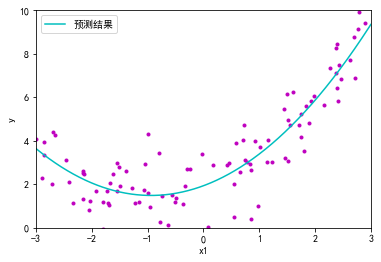当存在多个特征时，多项式回归能够发现特征和特征之间的关系（纯线性回归模型做不到这一点）。这是因为PolynomialFeatures会在给定的多项式阶数下，添加所有特征组合。当degree=d时，PolynomialFeatures可以将一个包含n个特征的数组转换为包含

n

+

d

d

!

n

!

\frac{n+d}{d!n!}

个特征的数组。要小心特征组合的数量爆炸！
3.学习曲线
高阶多项式回归对训练数据的拟合，很可能会比简单线性回归要好，但是也可能对训练数据过度拟合。那么怎么才能判断模型是过度拟合还是拟合不足呢？
如果使用交叉验证来评估模型的泛化性能。如果模型在训练集上表现良好，但是交叉验证的泛化表现非常糟糕，那么模型就是过度拟合。如果在二者上的表现都不佳，那就是拟合不足。这是判断模型太简单还是太复杂的一种方法。
还有一种方法是观察学习曲线：这个曲线绘制的是模型在训练集和验证集上，关于“训练集大小”的性能函数。要生成这个曲线，只需要在不同大小的训练子集上多次训练模型即可。下面这段代码，在给定训练集下定义了一个函数，绘制模型的学习曲线：
from sklearn.metrics import mean_squared_error
from sklearn.model_selection import train_test_split
def plot_learning_curves(model,X,y):
X_train,X_val,y_train,y_val=train_test_split(X,y,test_size=0.2)
train_errors,val_errors=[],[]
for m in range(1,len(X_train)):
model.fit(X_train[:m],y_train[:m])
y_train_predict=model.predict(X_train[:m])
y_val_predict=model.predict(X_val)
train_errors.append(mean_squared_error(y_train_predict,y_train[:m]))
val_errors.append(mean_squared_error(y_val_predict,y_val))
plt.plot(np.sqrt(train_errors),'r-+',linewidth=2,label='训练集')
plt.plot(np.sqrt(val_errors),'b-',linewidth=3,label='验证集')
plt.legend()
plt.xlabel('训练集大小')
plt.ylabel('RMSE')
plt.show()

看看纯线性回归模型（一条直线）的学习曲线：
lin_reg=LinearRegression()
plot_learning_curves(lin_reg,X,y)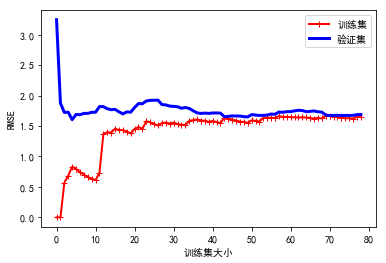这条学习曲线是典型的模型拟合不足。两条曲线均到达高地，非常接近，而且相当高。
如果模型对训练数据拟合不足，添加更多训练示例也于事无补。我们需要使用更复杂的模型或者找到更好的特征。
现在我们再来看看在同样的数据集上，一个10项多阶式模型的学习曲线：
from sklearn.pipeline import Pipeline
polynomial_regression=Pipeline((
('poly_features',PolynomialFeatures(degree=10,include_bias=False)),
('sgd_reg',LinearRegression()),
))
plt.ylim(0,3)
plot_learning_curves(polynomial_regression,X,y)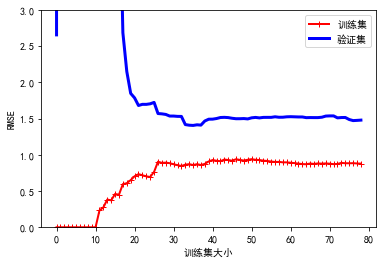这条学习曲线看起来跟眼前一条差不多，但是有两个非常大的区别：
训练数据的误差远低于线性回归模型。两条曲线之间有一定差距。这意味着该模型在训练数据上的表现比验证集上要好很多，这正是过度拟合的标志。但是，如果我们使用更大的训练集，那么这两条曲线将会越来越近。
改进模型过度拟合的方法之一是提供更多的训练数据，直到验证误差接近训练误差。
4.偏差/方差权衡
在统计学习和机器学习领域，一个重要的理论结果是，模型的泛化误差可以被表示为三个截然不同的误差之和：
偏差：这部分泛化误差的原因在于错误的假设，比如假设数据是线性的，而实际上是二次的。高偏差模型最有可能对训练数据拟合不足。方差：这部分误差是由于模型对训练数据的微小变化过度敏感导致的。具有高自由度的模型（例如高阶多项式模型）很可能也有高方差，所以很容易对训练数据过度拟合。不可避免的误差：这部分误差是因为数据本身的噪声所致。减少这部分误差的唯一方法就是清理数据（例如修复数据源，或者是检测并移除异常值）。
增加模型的复杂度通常会显著提升模型的方差，减少偏差。反过来，降低模型的复杂度则会提升模型的偏差，降低方差。这就是为什么称其为权衡。
展开全文机器学习
• 在一元线性回归中，输入特征只有一维， hθ=θ0+x1θ1h_{θ}=θ_0 + x_1θ_1, 对于多元特征，假设...对于非线性的一维数据，用线性回归拟合结果并不好，可以采用多项式回归，手动增加特征，例如如下4种多项式拟合 hθ
在一元线性回归中，输入特征只有一维，

hθ=θ0+x1θ1
$h_{θ}=θ_0 + x_1θ_1$, 对于多元特征，假设函数推广到了

hθ=θ0+x1θ1+x2θ2+x3θ3+...+xmθm
$h_{θ}=θ_0 + x_1θ_1+ x_2θ_2+ x_3θ_3+...+ x_mθ_m$  对于非线性的一维数据，用线性回归拟合结果并不好，可以采用多项式回归，手动增加特征，例如如下4种多项式拟合

hθ=θ0+θ1x1+θ2x21
$h_{θ}=θ_0 + θ_1x_1+θ_2x_1^2$ (1)

hθ=θ0+θ1x1+θ2x21θ2+θ3x31
$h_{θ}=θ_0 + θ_1x_1+θ_2x_1^2θ_2+θ_3x_1^3$ (2)

hθ=θ0+θ1x1+θ2log(x1)
$h_{θ}=θ_0 + θ_1x_1+θ_2log(x_1)$ (3)

hθ=θ0+θ1x1+θ2x√1
$h_{θ}=θ_0 + θ_1x_1+θ_2\sqrt x_1$ (4)  我们可以将多项式回归转换为多元回归，例如在式(1)中，可以令

x21
$x_1^2$为

x2
$x_2$，此时假设函数为

hθ=θ0+θ1x1+θ2x2
$h_{θ}=θ_0 + θ_1x_1+θ_2x_2$ ，  根据梯度下降求出

θ0，θ1，θ2
$θ_0，θ_1，θ_2$ 。  在上一篇博文一元线性回归中，  (http://blog.csdn.net/murphy_coolcoder/article/details/78946003)  最终拟合出的曲线如图所示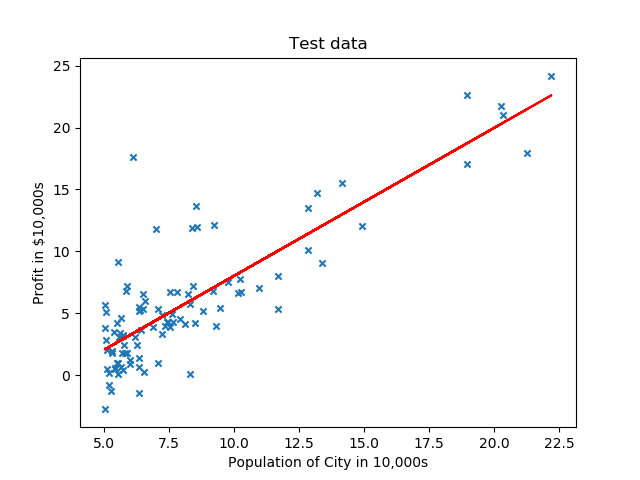如果我们想采用如下曲线拟合

hθ=θ0+θ1x1+θ2log(x1)
$h_{θ}=θ_0 + θ_1x_1+θ_2log(x_1)$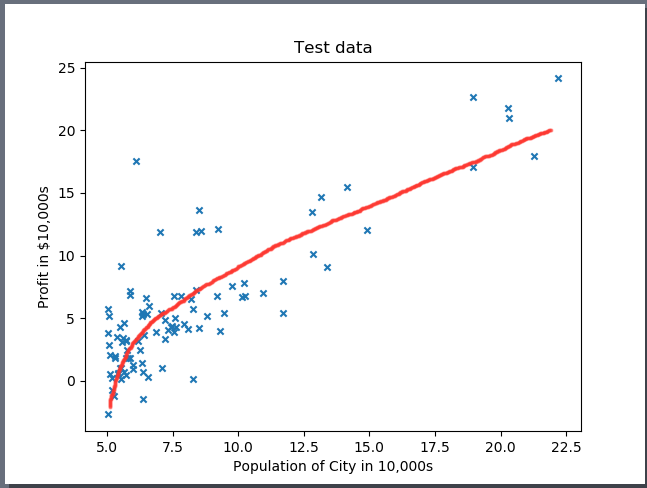或者如下二次函数拟合

hθ=θ0+θ1x1+θ2x21
$h_{θ}=θ_0 + θ_1x_1+θ_2x_1^2$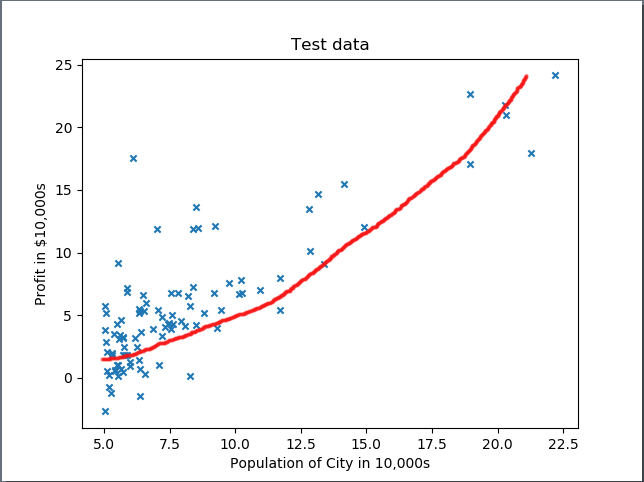则需要对输入数据增加一个对应的特征

X2
$X_2$, 若采用 log则

X2=log(X1)
$X_2 = log(X_1)$,若采用二次函数则

X2=X21
$X_2 = X_1^2$,然后进行梯度下降，得到

θ0，θ1，θ2
$θ_0，θ_1，θ_2$ 。  这里有几个trick要注意，  1).增加了一个特征之后，我们实际上将数据上升到3维空间，在做梯度下降之前，我们对训练样本先要进行归一化，尤其如果是高次多项式，特征之间数值差别很大，不做归一化梯度下降会很慢，而且还容易停止在局部最优而不是全局最优，不利于后续数值处理。  2).由于多项式回归是单一特征的多项式和，因此可以在二维空间展示出来，就如上图二所示。要在二维空间展示多项式曲线，对于输入数据要先做归一化，再增加特征向量。但此时会有一个问题存在，如果是多项式是log函数，在使用z-score归一化的情况下，没法在二维空间展示。因为采用z-score归一化的一维特征Norm_X_1$Norm_X_1$会有负值存在，此时在此基础之上增加log(Norm_X_1)$log(Norm_X_1)$特征会无效. 只能在三维空间展示。当然如果可以采用某种归一化方法，使其一维特征经过归一化后没有负值和0值存在，那么二维特征log(Norm_X_1)$log(Norm_X_1)$便可以运算，则也可以在二维空间展示出来，本文不做深入探讨。  3). 对于测试样本，测试样本的归一化参数要使用训练样本的归一化参数。如训练样本采用z-score归一化方法， 均值u1, 方差sigma1。 对于测试样本，测试样本的归一化参数不应使用自身的均值u2和方差sigma2而应使用训练样本的均值u1 和方差sigma1 作为归一化参数。  4). 在使用Matplotlib 绘制拟合函数时，建议使用散点图绘制，可以避免参数顺序变化带来的图像错乱。
源代码如下，数据集  训练样本数据https://pan.baidu.com/s/1nuM66mt  note： 本代码对数据集采用8：2的比例作为训练样本与测试样本，由于使用了np.random.shuffle()函数，每次运行的结果不尽相同，如要想要修改训练样本与测试样本的比例或者固定样本选择顺序，可以修改类中 sampleDivde 方法，参数“ratio”可以调整比例，注释掉np.random.shuffle()函数可以固定训练样本选择顺序。
import numpy as np
import matplotlib.pyplot as plt
from mpl_toolkits.mplot3d import Axes3D
from scipy.interpolate import spline

class LinearRegression:
def sampleDivde(self,data_X,data_Y,feature_num,ratio):
combineMatix = np.append(data_X.T,data_Y).reshape(feature_num+1,data_X.shape).T
np.random.shuffle(combineMatix)
m = int(data_X.shape*ratio)
train_X = combineMatix[:m,:feature_num]
train_Y = combineMatix[:m,feature_num]
test_X =combineMatix[m:,:feature_num]
test_Y = combineMatix[m:,feature_num]
return train_X,train_Y,test_X,test_Y
def featureScale(self, data_X):
u = np.mean(data_X,0)
sigma = np.std(data_X,0)
newData = (data_X - u)/sigma
return newData, u, sigma
def testScale(self,data_X,u,sigma):
newData = (data_X - u) / sigma
return newData

biasX = np.ones(data_X.shape)
newX = np.append(biasX, data_X.T).reshape(feature_num + 1, data_X.shape).T
return newX
if model == 0:
return data_X
if model == 1:
elif model == 2:
elif model == 3:
X = np.append(data_X, feature_add).reshape(feature_num + 1, data_X.shape).T
return X
def costFunc(self,theta,data_X,data_Y):
m = data_X.shape
J = np.dot((np.dot(theta,data_X.T).T- data_Y).T,np.dot(theta,data_X.T).T-data_Y)/(2*m)
return J
m = data_X.shape
for i in range(num_iters):
theta = theta - np.dot(data_X.T,np.dot(theta.T,data_X.T).T- data_Y)*alpha/m
return theta
def error(self,theta,test_X,Y_label):
test_Y = np.dot(theta,test_X.T)
error = sum(test_Y-Y_label)/Y_label.shape
return error
def learningRateCheck(self,theta,data_X,data_Y,alpha):
m = data_Y.shape
j_X = []
j_Y = []
for i in range(0,100,5):
j_X.append(i)
for j in range(i):
theta = theta - np.dot(data_X.T, np.dot(theta.T, data_X.T).T - data_Y) * alpha / m
j_Y.append(self.costFunc(theta,data_X,data_Y))
xNew = np.linspace(min(j_X), max(j_X), 300)
ySmooth = spline(j_X,j_Y,xNew)
plt.plot(xNew,ySmooth, color = 'red')
plt.show()
if __name__ =='__main__':
path = 'Housedata.txt'
fr = open(path, 'r+')
x = []
y = []
for line in fr:
items = line.strip().split(',')
x.append(float(items))
y.append(float(items))
test = LinearRegression()
data_X = np.array(x)
data_Y = np.array(y)
a = data_X
z = data_Y
train_X, train_Y, test_X, test_Y = test.sampleDivde(data_X, data_Y,1,0.8) #no bias
#Linear regression data pre-processing
norm_train_X,u,sigma = test.featureScale(train_X)
norm_test_X = test.testScale(test_X,u,sigma)
theta = np.zeros(biasnorm_train_X.shape)
theta_Results = test.gradientDescent(theta, biasnorm_train_X, train_Y, 0.1, 1500)
#Plot data
X = train_X
Y = np.dot(theta_Results,  biasnorm_train_X.T)
plt.subplot(211)
plt.plot(X, Y, color='red')
plt.scatter(data_X, data_Y, s=20, marker="x") #plot raw data
plt.title('Linear fit')

#Polyomial Regression#
# train data used for train
# test data used for train
theta = np.zeros(Q_biasnorm_train_X.shape)
Q_theta_Results = test.gradientDescent(theta, Q_biasnorm_train_X, train_Y, 0.1, 1500)
#data used for plot,which is featureScale first, than add feature X2.
Q_norm_X,p_u,p_sigma = test.featureScale(train_X)
# Plot data
X = train_X
Y = np.dot(Q_theta_Results, Q_biasnorm_X.T)
plt.subplot(212)
plt.scatter(X, Y, color='red')
plt.scatter(data_X, data_Y, s=20, marker="x")  # plot raw data
plt.show()

# ************3. Log fit*********
# train data used for train
# test data used for train
theta = np.zeros(L_biasTrain_X.shape)
L_theta_Results = test.gradientDescent(theta, L_biasTrain_X, train_Y, 0.2, 1500)
# Plot 3D data
ax = plt.subplot(111, projection='3d')
X = train_X
Y = np.log2(train_X)
Z = np.dot(L_theta_Results, L_biasTrain_X.T)
ax.scatter(X,Y,Z, color='g')
ax.scatter(X,Y,train_Y, c='r')  # 绘制数据点
ax.set_zlabel('Z')  # 坐标轴
ax.set_ylabel('Y')
ax.set_xlabel('X')
plt.show()

######Error  check########
error = test.error(theta_Results, biastest_X, test_Y)
print("Linear regression error is:",error)
Q_error = test.error(Q_theta_Results, Q_bias_test_X, test_Y)
L_error = test.error(L_theta_Results, L_biasTest_X, test_Y)
print("Log Polyomial Regression error is:",L_error)
二次拟合结果与一元线性回归拟合比较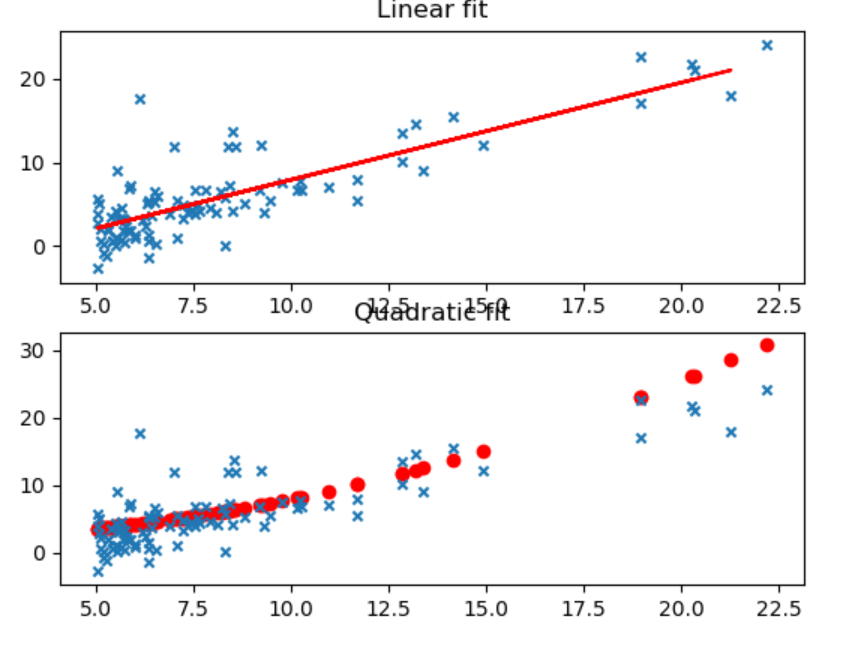Log 拟合三维图像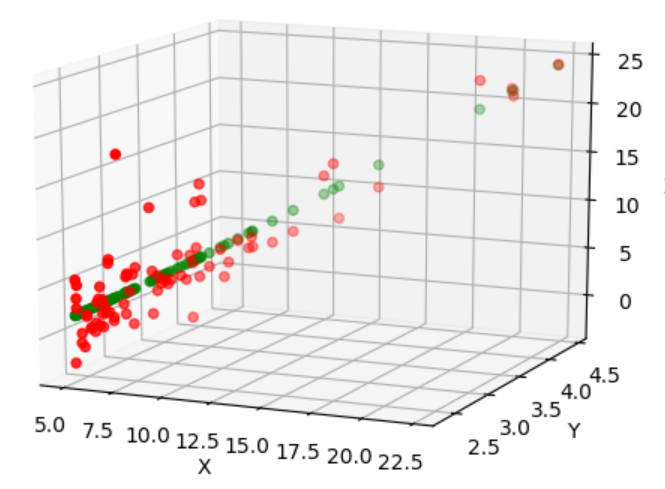拖动三维图像，可以看出X与Y 的对数关系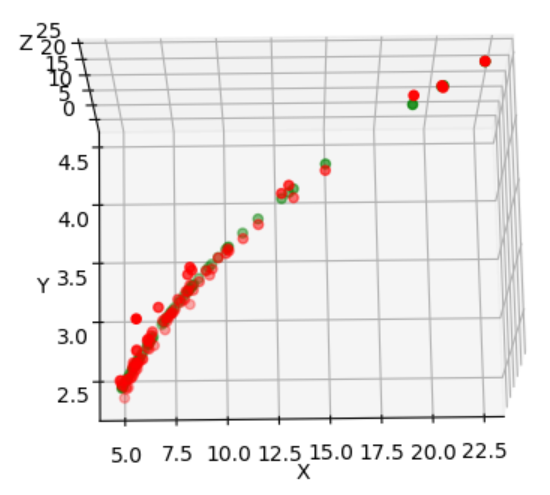由于采取了随机策略，由于训练样本选择的不同，三种方法的误差比较也不尽相同。某些情况下Log拟合最优，某些情况下线性回归反而表现要好。这是与数据集分不开的。就本实例的数据集来说，三种拟合方式差别不大。对应于不同的数据集，采用不同的方式，甚至更高次的多项式拟合，要根据具体问题来分析。本文只是给简要分析了一下多项式拟合和多元线性回归二者之间的关系以及我在调试代码过程中所遇到的问题，以供参考。
展开全文• 单变量线性回归（Linear Regression with One Variable） 预测器表达式： 选择合适的参数（parameters）θ0 θ1，其决定了直线相对于训练集的准确程度。 建模误差（modeling error）：训练集中，...
• 目录6.0 导言6.1 一维核光滑器6.1.1 局部线性回归6.1.2 局部多项式回归6.2 选择核宽度6.3 Rp\mathbb R^pRp中的局部回归 6.0 导言 P192 本章的方法都是memory-based，很少或不需要训练。模型就是整个训练集（PRML中...机器学习 算法 核函数 数学
• #非线性回归模型 setwd("I:/研一课程/2.2回归分析/R/data")#设定当前的工作目录，重要！ a a.nls a.sum a.sum #（1）采用龚珀兹模型形式，建立非线性模型 SST Rsquare Rsquare #多项式回归 setwd("I:/...
• 1.什么是线性回归线性回归是试图在一堆数据中训练得到自变量x因变量y中一组...在统计学中，线性回归是利用称为线性回归方程的最小二乘函数对一个或多个自变量因变量之间关系进行建模的一种回归分析。 周志...机器学习
• 总览 在这里，我们放宽了流行的线性技术的线性假设。有时线性假设只是一个很差的近似值。有许多方法可以解决此问题，其中一些方法可以通过使用正则化... 多项式回归 这是对数据提供非线性拟合的简单方法。 阶...R语言 平滑样条
• ## 断点分析法_局部线性回归_matlab

万次阅读 热门讨论 2016-07-11 10:51:58
本文介绍断点分析法在数据作假方面的应用 局部线性回归的matlab实现局部加权线性回归 断点分析法 matlab 核函数
• 1.什么是线性回归线性回归是试图在一堆数据中训练得到自变量x因变量y中一组线性关系，如。例如把人脚底板长度作为自变量，身高作为因变量，那么在这两种数据之间就可以做一个简单线性回归，可...
• 线性回归虽然简单，但是容易出现问题：就是“欠拟合”“过拟合”，欠拟合是由于我们并不能很好的拟合我们的训练数据，导致出现较大的训练误差；而过拟合是由于我们过度拟合训练数据，导致我们的模型过度复杂而产生...欠拟合
• 前面博客有讲到，样本如果不是线性的可以通过多项式扩展，映射到多维空间来拟合。如此之外，还可以做一个局部加权线性回归（Locally Weighted Linear Regression，LWLR）。 ...
• 局部加权回归的方法如下，首先看线性多项式回归的损失函数 很明显，局部加权回归在每一次预测新样本时都会重新确定参数，以达到更好的预测效果。当数据规模比较大的时候计算量很大，学习效率很低。并且局部加权...
• 书籍：《机器学习实战》中文版 ...一、一般线性回归（最小二乘法OLS） 回归系数求解公式： 说明：X矩阵中每一行是一个样本，y是列向量。只有逆矩阵存在的时候使用，必须在代码中进行判断。 from numpy机器学习实战
• 本文主要总结了解决非线性回归问题的机器学习方法，其中包括多项式线性模型、广义线性(GAM)模型、回归树模型、支持向量回归(SVR)模型，每个模型的方法都有其特点。 多项式线性模型GAM模型侧重于经验风险误差最小...GAM模型 回归树模型 SVR模型
• 1. 核平滑方法 代码实现 2. 局部多项式回归 2.1 加权最小二乘法(Weighted least squares) 2.2 局部多项式回归(Local polynomial kernel regression) 代码实现
• 为了更好地拟合颜色空间之间复杂的映射关系，在自适应局部线性回归颜色校正模型的基础上提出了基于自适应局部非线性回归的颜色校正模型，在小样本情况下，自适应地选择插值点的个数。利用局部非线性回归模型优化权值...
• 多项式回归 扩展可能是假设某些多项式函数， 同样，在标准线性模型方法（使用GLM的条件正态分布）中，参数可以使用最小二乘法获得，其中在。 即使此多项式模型不是真正的多项式模型，也可能仍然是一个很好的...R语言 核平滑
• 一 概述通常情况下的线性拟合不能很好地预测所有的值，因为它容易导致欠拟合（under ...局部加权回归（LWR）是非参数学习方法。 首先参数学习方法是这样一种方法：在训练完成所有数据后得到一系列训练参数，然后根据训机器学习
• 局部多项式回归拟合是对两维散点图进行平滑的常用方法，它结合了传统线性回归的简洁性线性回归的灵活性。当要估计某个响应变量值时，先从其预测变量附近取一个数据子集，然后对该子集进行线性回归或二次回归，...
• 通常情况下的线性拟合不能很好地预测所有的值，因为它容易导致欠拟合（under fitting），比如数据集是 一个钟形的曲线。而多项式拟合能拟合所有数据，但是在预测新样本的时候又会变得很糟糕，因为它导致数据的 ...机器学习
• #初始化线性回归器 ] ) polynomial_regression . fit ( X , y ) #训练模型 y_newbig = polynomial_regression . predict ( X_new ) #预测数据 plt . plot ( X_new , y_newbig , style , label = ...python
• 由拟合问题，突出特征选择的重要性。局部权重线性回归在数据充足的情况下，可缓解特征选择问题。而后详述特征选择的步骤相关参数。非参数算法 特征选择
• 局部加权线性回归（LWLR）对于线性回归算法，容易出现欠拟合，而多项式回归又容易出现过拟合。因此出现了局部加权回归模型y(i)=θT⋅x(i) y^{(i)}=\theta^T \cdot x^{(i)} 和线性回归的模型相同，但是对于每一个预测...
• 一元线性回归：梯度下降法 一元线性回归线性回归的最简单的一种，即只有一个特征变量。首先是梯度下降法，这是比较经典的求法。一元线性回归通俗易懂地说，就是一元一次方程。只不过这里的斜率截距要通过最小...机器学习...